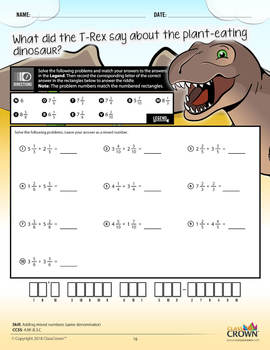# 4th Grade Math Worksheets – Fourth Grade Math Pack 2 - Math Riddles - CCSS4th, Homeschool
Subjects
Standards
Resource Type
Formats Included
• PDF
Pages
51 pages

\$20.99
\$26.97
Save \$5.98

### Description

Math Series: Riddle-Me-Worksheets
4th Grade – Pack 2 (Multi-Skill)

Do your students ever complete an entire worksheet, only to find that they have done most of the problems incorrectly and clearly have a misconception?

ClassCrown’s Riddle-Me-Worksheets help to prevent this problem by introducing a puzzle aspect to math, giving students immediate feedback as to whether or not they are solving problems correctly. If the answer to the riddle isn't correct, the student knows which problems he's made an error on.

This 4th grade pack 2 contains skills covered in fourth grade math. While some of the included skills may be advanced for a younger student, the more difficult pages can be used as enrichment for advanced students. Each page has been designed in full, vibrant color for maximum quality but has been engineered to look great when printed in black and white.

Skill Page Count: 30
Math Skill: Multiple Skills / Grade 4 Math (Pack 2)

Skills Included:
1 – Finding equivalent fractions

CCSS: 4.NF.A.1

2 – Finding equivalent fraction patterns

CCSS: 4.NF.A.1

3 – Simplifying fractions

CCSS: 4.NF.A.1

4 – Comparing fractions

CCSS: 4.NF.A.2

5 – Comparing fractions to one half

CCSS: 4.NF.A.2

6 – Adding fractions - visual

CCSS: 4.NF.B.3.B

7 – Adding parts of a whole - visual

CCSS: 4.NF.B.3.B

8 – Adding fractions - numeric & visual

CCSS: 4.NF.B.3.B

9 – Adding mixed numbers - visual

CCSS: 4.NF.B.3.C

10 – Adding and subtracting improper fractions - same denominator

CCSS: 4.NF.B.3.C

11 – Adding mixed numbers - same denominator

CCSS: 4.NF.B.3.C

12 – Converting an improper fraction to a mixed number

CCSS: 4.NF.B.3.C

13 – Converting a mixed number to an improper fraction

CCSS: 4.NF.B.3.C

14 – Subtracting fractions with regrouping - same denominator

CCSS: 4.NF.B.3.C

15 – Adding and subtracting fractions, word problems - same denominator

CCSS: 4.NF.B.3.D

16 – Multiplying fractions by whole numbers - visual

CCSS: 4.NF.B.4.A

17 – Multiplying fractions by whole numbers - visual, bar

CCSS: 4.NF.B.4.A

18 – Multiplying unit fractions with a number line

CCSS: 4.NF.B.4.A

19 – Creating equivalent unit fraction problems

CCSS: 4.NF.B.4.B

20 – Multiplying fractions by whole numbers - word problems

CCSS: 4.NF.B.4.C

21 – Expressing a fraction with a denominator 10 as a fraction with denominator 100

CCSS: 4.NF.C.5

22 – Finding decimals on a number line

CCSS: 4.NF.C.5

23 – Converting decimals to fractions

CCSS: 4.NF.C.6

24 – Finding equivalent fractions and decimals - visual

CCSS: 4.NF.C.6

25 – Converting fractions to decimals - tenths and hundreds

CCSS: 4.NF.C.6

26 – Comparing decimals

CCSS: 4.NF.C.6

27 – Determining greatest and least value

CCSS: 4.NF.C.7

28 – Ordering decimals

CCSS: 4.NF.C.7

29 – Ordering money, same dollar amount

CCSS: 4.NF.C.7

30 – Ordering money, different dollar amounts

CCSS: 4.NF.C.7

Features:
• High Quality PDF format
• Full vibrant color pages that print great in black and white
• Common Core aligned

Feel free to let us know how we're doing in the Ratings & Comments section. Also, check us out on the web at www.classcrown.com and don't forget to follow us on twitter for updates and teacher information.

---------------------------------------------------------------------------------------
The contents of this document are copyrighted by ClassCrown™.

The redistribution, resale, modification, or republication of any part of this document and its attachments is strictly prohibited. See terms of use below.

Personal Use: With your purchase, you are being granted permission to print the digital work. All copyrights remain with © 2018 ClassCrown.
---------------------------------------------------------------------------------------

Total Pages
51 pages
Included
Teaching Duration
N/A
Report this Resource to TpT
Reported resources will be reviewed by our team. Report this resource to let us know if this resource violates TpT’s content guidelines.

### Standards

to see state-specific standards (only available in the US).
Explain why a fraction 𝘢/𝘣 is equivalent to a fraction (𝘯 × 𝘢)/(𝘯 × 𝘣) by using visual fraction models, with attention to how the number and size of the parts differ even though the two fractions themselves are the same size. Use this principle to recognize and generate equivalent fractions.
Compare two fractions with different numerators and different denominators, e.g., by creating common denominators or numerators, or by comparing to a benchmark fraction such as 1/2. Recognize that comparisons are valid only when the two fractions refer to the same whole. Record the results of comparisons with symbols >, =, or <, and justify the conclusions, e.g., by using a visual fraction model.
Understand a fraction 𝘢/𝘣 with 𝘢 > 1 as a sum of fractions 1/𝘣.
Decompose a fraction into a sum of fractions with the same denominator in more than one way, recording each decomposition by an equation. Justify decompositions, e.g., by using a visual fraction model. Examples: 3/8 = 1/8 + 1/8 + 1/8; 3/8 = 1/8 + 2/8; 2 1/8 = 1 + 1 + 1/8 = 8/8 + 8/8 + 1/8.
Add and subtract mixed numbers with like denominators, e.g., by replacing each mixed number with an equivalent fraction, and/or by using properties of operations and the relationship between addition and subtraction.